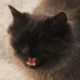# Variable Line Resolution #2

Actionscript:
1. var loc:Vector.<Point> = new Vector.<Point>();
2. var lifts:Vector.<int> = new Vector.<int>();
3. var index:int = 0;
4. var resolution:int = 1;
5. var down:Boolean;
10. function onKeyReleased(evt:KeyboardEvent):void{
11.     if (evt.keyCode == Keyboard.RIGHT){
12.         resolution -= 1;
13.         if (resolution <1) resolution = 1;
14.     }else
15.     if (evt.keyCode == Keyboard.LEFT){
16.         resolution += 1;
17.     }
18. }
19. function onDown(evt:MouseEvent):void{
20.     down = true;
21.     lifts.push(index);
22. }
23. function onUp(evt:MouseEvent):void{
24.     down = false;
25. }
26. function onLoop(evt:Event):void {
27.     if (down){
28.         loc[index] = new Point(mouseX, mouseY);
29.         index++;
30.     }
31.     graphics.clear();
32.     graphics.lineStyle(0,0x000000);
33.     var j:int = 0;
34.     var lift:int;
35.     var liftLength:int = lifts.length;
36.     var lastLoc:int =  loc.length  - 1;
37.     for (var i:int = 0; i<liftLength; i++){
38.         j =  lifts[i];
39.         graphics.moveTo(loc[j].x, loc[j].y);
40.         if (i <liftLength - 1){
41.             lift = lifts[i + 1] - 1;
42.         }else{
43.             lift = lastLoc;
44.         }
45.         while (j <lift){
46.             j++;
47.             if (j % resolution == 1 || resolution == 1){
48.               graphics.lineTo(loc[j].x, loc[j].y);
49.             }
50.         }
51.         graphics.lineTo(loc[j].x, loc[j].y);
52.     }
53. }

This snippet shows a simple approach to creating variable resolution graphics.

This is an improved version of yesterdays post. In yesterdays example the end points of the lines would sometimes be removed... this version doesn't have that problem...

Below is a drawing created using this snippet. The left arrow key is used to decrease resolution and the right arrow key is used to increase resolution:

Have a look at the swf...1.Lawrie
Posted July 9, 2009 at 2:40 am | Permalink

Hi Zevan,
Another great snippet here - I think it would look awesome to have a set of images loading in, with a very low resolution and have them gradually animate to their high resolution -I think it would give the effect that the computer was procedurally drawing them…

2.Zevan
Posted July 9, 2009 at 9:44 am | Permalink

Thanks Lawrie… yeah there are definitely some interesting possibilities with this…